# Invariant

(diff) ← Older revision | Latest revision (diff) | Newer revision → (diff)

A mappingof a given collectionof mathematical objects endowed with a fixed equivalence relation, into another collectionof mathematical objects, that is constant on the equivalence classes ofwith respect to(more precisely, that is an invariant of the equivalence relationon). Ifis an object in, then one often says thatis an invariant of the object. The concept of an invariant is one of the most important in mathematics, since the study of invariants is directly related to problems of classification of objects of some type or other. Essentially, the aim of every mathematical classification is to construct some complete system of invariants (if possible, one as simple as possible), that is, a system that distinguishes any two inequivalent objects of the collection under consideration.

The simplest examples of invariants are the invariants of the real plane second-order curves (cf. Second-order curve). Thus, letbe the set of all such non-splitting curves and letbe the equivalence relation ongiven by the rule: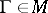is equivalent to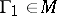if and only if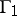is obtained fromby a motion (that is, an isometry, cf. Isometric mapping) of the plane. If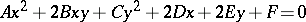is the equation of the curvein a Cartesian coordinate system, let,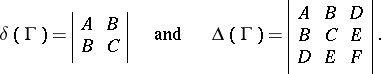Then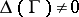and the numbers,do not depend on the choice of the coordinate system (even though the equation ofitself does depend on it). If two curvesare equivalent, thenand. In other words, the mappingsandfrom the setinto the setof all real numbers are invariants of the equivalence relation; these mappings are also called invariants of real plane second-order non-splitting curves. The values of these invariants on a specific curve enable one to determine the type of this curve (ellipse, hyperbola, parabola).

Another classical example is the cross ratio of an ordered set of four points lying on a real projective line. The cross ratio does not change if these points undergo a projective transformation of the line. In this example:is the set of ordered quadruples of points of a real projective line; the equivalence relationonis defined by the rule: two sets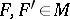are equivalent if and only ifis taken into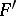by a projective transformation of the line; andis the set of real numbers completed by infinity. Taking the cross ratio defines a mapping fromintothat is an invariant of the relation; it is in this sense that one says that the cross ratio is an invariant of four points (with respect to the projective group).

Associating to a quadratic form invariables its rank also gives an example of an invariant: the rank does not change when the form is replaced by an equivalent one (for short, the rank is an invariant of quadratic forms). Furthermore, if the forms are considered over the field of complex numbers, then the rank constitutes a complete system of invariants of forms invariables; two forms are equivalent if and only if they have the same rank. If, on the other hand, one considers forms over the field of real numbers, then there arises another invariant, namely, the signature of the form; rank and signature constitute a complete system of invariants. In these examples,is the set of quadratic forms invariables,is the equivalence relation defined by non-singular linear transformation of the variables andis the set of integers.

The common feature uniting these (and many other) examples is that the equivalence relationis defined by some groupof transformations of the set(that is,are-equivalent if and only if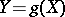for some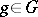). The invariants arising in such cases are called invariants of the group. In the first example, these are the transformations ofinduced by the group of isometries of the plane, in the second, by the projective group, and in the third, by the general linear group of non-singular transformations of the variables. These examples illustrate the general concept, advanced by F. Klein (the so-called Erlangen program), according to which each group of transformations can serve as the group of "transformations of a coordinate system" (automorphisms) in some geometry; the quantities defined by the objects of this geometry that do not change under a "coordinate change" (the invariants) describe the intrinsic properties of the geometry under consideration and provide the "structural" classification of its theorems. For example, the problem of projective geometry is to find invariants (and relations between them) for the projective group; for Euclidean geometry, for the group of motions (isometries) of Euclidean space, etc.

In this way the classical theory of invariants (cf. Invariants, theory of) was developed, in which only invariants of special type are considered (namely, polynomial or rational invariants for groups of linear transformations or, more broadly, numerical functions that are constant on the orbits of some group).

However, the more general concept of an invariant is a broader one and need not be restricted within the framework of invariants of a transformation group, since not every equivalence relationon a setof mathematical objects under consideration is determined by a group action. Examples of invariants of such a type can be given in many areas of mathematics. In algebraic topology and homotopic topology one associates to each topological space its homotopy groups as well as its singular homology groups (with coefficients in some group); these groups are invariant with respect to homotopy equivalence of spaces. In algebraic geometry one considers the relation of birational equivalence of algebraic varieties; the dimension of a variety and, if one restricts oneself to smooth complete varieties — the arithmetic genus, provide an example of invariants of this equivalence relation. In differential topology manifolds are considered up to diffeomorphisms; the Stiefel–Whitney classes of a manifold are invariant with respect to this equivalence relation. In classical differential geometry one considers the integral curvature of a closed surface; this is a bending invariant. In the theory of Abelian groups one considers so-called invariants of finitely-generated groups, namely the rank and the orders of the primary components; these constitute a complete set of invariants for the set of such groups, considered up to isomorphism.